## Welcome to the Second Life Forums Archive

These forums are CLOSED. Please visit the new forums HERE

# Discussion: peedometer Working By Hypottinuse

Jiminy Roo
Registered User
Join date: 25 Dec 2004
Posts: 42
01-16-2005 01:50
Here is a Speedometer I made this morning, because I got bored. It will Give your speed in KPH, rounded to the nearest KPH. It works by subtracting the x, y, z values of the current pos, and the pos from 0.5 seconds ago. it then works out the horizontal distance traveled,

square root of (square root of( (x1*x2) + (y1*y2) ) * square root of ( z1 * z2 )))

And here is the code:
CODE
`vector current;vector new;float xvalue;float yvalue;float zvalue;default{    state_entry()    {        vector current = llGetPos();        llSetTimerEvent(0.5);    }        timer()    {        new = llGetPos();        if ( new. x < current .x)        { xvalue = current .x - new .x;        }        if ( new .x > current .x)        {         xvalue =  new .x - current .x ;        }          if ( new .x == current .x)        {         xvalue =  new .x - current .x ;        }                                if ( new.y < current .y)        { yvalue = current .y  - new .y  ;        }        if ( new .y > current .y)        {         yvalue =  new .y - current .y ;        }         if ( new .y == current .y)        {         yvalue =  new .y - current .y ;        }                                        if ( new.z < current .z)        { zvalue = current .z  - new .z  ;        }        if ( new .z > current .z)        {         zvalue =  new .z - current .z ;        }         if ( new .z == current .z)        {         zvalue =  new .z - current .z ;        }                float beforevalue = llSqrt((yvalue * yvalue) + (xvalue * xvalue));        float aftervalue =  llSqrt((beforevalue * beforevalue) + (zvalue * zvalue));                llSetText((string)llRound((7.2 * aftervalue)) + " kph", <1,0,0>, 1);        current = new;    } }`
Kwipper Manimal
Registered User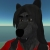Join date: 12 Jan 2005
Posts: 52
04-28-2006 10:50
Awesome script, but one question. If I wanted to convert this to Miles Per Hour instead of Kilometers Per Hour, what would the script be then?
Nada Epoch
The Librarian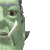Join date: 4 Nov 2002
Posts: 1,423
Original Thread
04-28-2006 21:30
/15/99/33103/1.html
_____________________
i've got nothing.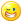Nada Epoch
The LibrarianJoin date: 4 Nov 2002
Posts: 1,423
04-28-2006 21:36
change this line
CODE
`        llSetText((string)llRound((7.2 * aftervalue)) + " kph", <1,0,0>, 1);`

to
CODE
`        llSetText((string)llRound((7.2 * aftervalue * 0.62)) + " mph", <1,0,0>, 1);`
_____________________
i've got nothing.Kwipper Manimal
Registered UserJoin date: 12 Jan 2005
Posts: 52
04-29-2006 04:37
Hey thanks! =)

BTW. I noticed something. I have always suspected that the avatars always walked a lot faster than they should have.. realistically.. and now I got proof.

The average human being usually walks about 2 - 3 mph on average and acording to this readout. I am getting a 6 - 7 mph average walking speed in Second Life.
Kyrah Abattoir
cruelty delight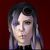Join date: 4 Jun 2004
Posts: 2,786
04-29-2006 06:23
you will call me mean but... what about llGetVel() ?
_____________________tired of XStreetSL? try those!
apez http://tinyurl.com/yfm9d5b
metalife http://tinyurl.com/yzm3yvw
metaverse exchange http://tinyurl.com/yzh7j4a
slapt http://tinyurl.com/yfqah9u
Kwipper Manimal
Registered UserJoin date: 12 Jan 2005
Posts: 52
04-29-2006 07:28
Uhh... what about it?
Prometheus Deckard
Registered User
Join date: 24 May 2005
Posts: 23
04-29-2006 14:22
Couldn't you use llVecMag(llGetVel()); to calculate your speed?
Ordinal Malaprop
really very ordinary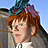Join date: 9 Sep 2005
Posts: 4,607
04-29-2006 14:28
It would only trigger whenever the timer triggered, though. That wouldn't make any significant difference overall I suppose, given that it's a rapid timer averaged over a long period and most people don't stop and start moving within half a second.
Francis Chung
This sentence no verb.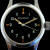Join date: 22 Sep 2003
Posts: 918
05-01-2006 18:16
llGetVel() has a bug -

when you're sitting (ie. in a vehicle) and you cross a simline, attachments using llGetVel() might start reporting fictional results, like saying that you're travelling 50mph when you're standing still.

This can be inconvenient if you're trying to make a speedometer HUD.

It's been broken since at least SL 1.6 when I've noticed this bug. I've reported it, but LL doesn't seem to consider this an important enough bug to fix.

The above solution is a valid work-around for this bug. The Dominus Shadow uses a variation on this technique, but adds an exponentially weighted decay to smooth out the imprecision of the timer.
_____________________
--
~If you lived here, you would be home by now~
Myrilla Vixen
Definitely Bloo
Join date: 11 Jun 2005
Posts: 143
05-01-2006 18:50
Hey guys I probably did this awfully but then again I've never been good at teasing scripts others made..

I used the above script to make an avatar speed sensor for people around me, and it seems to have.. gone.. badsies.

If anyone wants to tell me how horribly I mangled this and how to make it better I'd appreciate it.

CODE
`vector current;vector new;float xvalue;float yvalue;float zvalue;string name0;string name1;string name2;string name3;string name4;string name5;string name6;string name7;string name8;string name9;string speed0;string speed1;string speed2;string speed3;string speed4;string speed5;string speed6;string speed7;string speed8;string speed9;default{    state_entry()    {        vector current = llGetPos();        llSensorRepeat("", NULL_KEY, AGENT, 30, PI, 0.5);    }        sensor(integer num_det)    {        integer x;        for(x = 0; x <= 9; x = ++x)        {            new = llDetectedPos(x);                    if ( new. x < current .x)            {                xvalue = current .x - new .x;            }                    if ( new .x > current .x)            {                xvalue =  new .x - current .x ;            }                        if ( new .x == current .x)            {                xvalue =  new .x - current .x ;            }                    if ( new.y < current .y)            {                yvalue = current .y  - new .y  ;            }                        if ( new .y > current .y)            {                yvalue =  new .y - current .y ;            }                    if ( new .y == current .y)            {                yvalue =  new .y - current .y ;            }                        if ( new.z < current .z)            {                zvalue = current .z  - new .z  ;            }                    if ( new .z > current .z)            {                zvalue =  new .z - current .z ;            }                        if ( new .z == current .z)            {                zvalue =  new .z - current .z ;            }                    float beforevalue = llSqrt((yvalue * yvalue) + (xvalue * xvalue));            float aftervalue =  llSqrt((beforevalue * beforevalue) + (zvalue * zvalue));                        if (x == 0)            {                speed0 = (string)llRound((7.2 * aftervalue));                name0 = llDetectedName(x);            }                        if (x == 1)            {                speed1 = (string)llRound((7.2 * aftervalue));                name1 = llDetectedName(x);            }                        if (x == 2)            {                speed2 = (string)llRound((7.2 * aftervalue));                name2 = llDetectedName(x);            }                        if (x == 3)            {                speed3 = (string)llRound((7.2 * aftervalue));                name3 = llDetectedName(x);            }                        if (x == 4)            {                speed4 = (string)llRound((7.2 * aftervalue));                name4 = llDetectedName(x);            }                        if (x == 5)            {                speed5 = (string)llRound((7.2 * aftervalue));                name5 = llDetectedName(x);            }                        if (x == 6)            {                speed6 = (string)llRound((7.2 * aftervalue));                name6 = llDetectedName(x);            }                        if (x == 7)            {                speed7 = (string)llRound((7.2 * aftervalue));                name7 = llDetectedName(x);            }                        if (x == 8)            {                speed8 = (string)llRound((7.2 * aftervalue));                name8 = llDetectedName(x);            }                        if (x == 9)            {                speed9 = (string)llRound((7.2 * aftervalue));                name9 = llDetectedName(x);            }                        current = new;        }                llSetText(name0 + ": " + speed0 + " kph \n" + name1 + ": " + speed1 + " kph \n" + name2 + ": " + speed2 + " kph \n" + name3 + ": " + speed3 + " kph \n", <1,0,0>, 1);                //Haven't put in all the lines yet, just the first 4 detected to test.    } }`

Edit: oh right! The problem is that it is giving false data, or at least data that I don't want.. closest avatar is listed as nearly 1800 kph and everyone else hangs just under 40kph when they stand still x.x;

Edit2: ok.. I kind get it.. it's comparing people to the position of the person next to them.. now I am trying to work around it >.>

Edit3: Ok, now everyone is listed as near to 1800kph, posting that code and hoping someone gets back to me with a simpler, more smarty solution.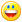CODE
`vector current;vector new;float xvalue;float yvalue;float zvalue;vector current0;vector current1;vector current2;vector current3;vector current4;vector current5;vector current6;vector current7;vector current8;vector current9;vector new0;vector new1;vector new2;vector new3;vector new4;vector new5;vector new6;vector new7;vector new8;vector new9;string name0;string name1;string name2;string name3;string name4;string name5;string name6;string name7;string name8;string name9;string speed0;string speed1;string speed2;string speed3;string speed4;string speed5;string speed6;string speed7;string speed8;string speed9;default{    state_entry()    {        vector current = llGetPos();        llSensorRepeat("", NULL_KEY, AGENT, 30, PI, 0.5);    }        sensor(integer num_det)    {        integer x;        for(x = 0; x <= 9; x = ++x)        {            new = llDetectedPos(x);                        if (x == 0)            {                current = new0;            }                        if (x == 1)            {                current = new1;            }                        if (x == 2)            {                current = new2;            }                        if (x == 3)            {                current = new3;            }                        if (x == 4)            {                current = new4;            }                        if (x == 5)            {                current = new5;            }                        if (x == 6)            {                current = new6;            }                        if (x == 7)            {                current = new7;            }                        if (x == 8)            {                current = new8;            }                        if (x == 9)            {                current = new9;            }                        //Sanity Break                    if ( new. x < current .x)            {                xvalue = current .x - new .x;            }                    if ( new .x > current .x)            {                xvalue =  new .x - current .x ;            }                        if ( new .x == current .x)            {                xvalue =  new .x - current .x ;            }                    if ( new.y < current .y)            {                yvalue = current .y  - new .y  ;            }                        if ( new .y > current .y)            {                yvalue =  new .y - current .y ;            }                    if ( new .y == current .y)            {                yvalue =  new .y - current .y ;            }                        if ( new.z < current .z)            {                zvalue = current .z  - new .z  ;            }                    if ( new .z > current .z)            {                zvalue =  new .z - current .z ;            }                        if ( new .z == current .z)            {                zvalue =  new .z - current .z ;            }                    float beforevalue = llSqrt((yvalue * yvalue) + (xvalue * xvalue));            float aftervalue =  llSqrt((beforevalue * beforevalue) + (zvalue * zvalue));            //Sanity Break                                    if (x == 0)            {                speed0 = (string)llRound((7.2 * aftervalue));                name0 = llDetectedName(x);                current0 = new0;            }                        if (x == 1)            {                speed1 = (string)llRound((7.2 * aftervalue));                name1 = llDetectedName(x);                current1 = new1;            }                        if (x == 2)            {                speed2 = (string)llRound((7.2 * aftervalue));                name2 = llDetectedName(x);                current2 = new2;            }                        if (x == 3)            {                speed3 = (string)llRound((7.2 * aftervalue));                name3 = llDetectedName(x);                current3 = new3;            }                        if (x == 4)            {                speed4 = (string)llRound((7.2 * aftervalue));                name4 = llDetectedName(x);                current4 = new4;            }                        if (x == 5)            {                speed5 = (string)llRound((7.2 * aftervalue));                name5 = llDetectedName(x);                current5 = new5;            }                        if (x == 6)            {                speed6 = (string)llRound((7.2 * aftervalue));                name6 = llDetectedName(x);                current6 = new6;            }                        if (x == 7)            {                speed7 = (string)llRound((7.2 * aftervalue));                name7 = llDetectedName(x);                current7 = new7;            }                        if (x == 8)            {                speed8 = (string)llRound((7.2 * aftervalue));                name8 = llDetectedName(x);                current8 = new8;            }                        if (x == 9)            {                speed9 = (string)llRound((7.2 * aftervalue));                name9 = llDetectedName(x);                current9 = new9;            }        }                llSetText(name0 + ": " + speed0 + " kph \n" + name1 + ": " + speed1 + " kph \n" + name2 + ": " + speed2 + " kph \n" + name3 + ": " + speed3 + " kph \n", <1,0,0>, 1);                    } }`
Argent Stonecutter
Emergency Mustelid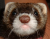Join date: 20 Sep 2005
Posts: 20,263
05-03-2006 12:20
From: Jiminy Roo
Here is a Speedometer I made this morning, because I got bored. It will Give your speed in KPH, rounded to the nearest KPH. It works by subtracting the x, y, z values of the current pos, and the pos from 0.5 seconds ago. it then works out the horizontal distance traveled,

square root of (square root of( (x1*x2) + (y1*y2) ) * square root of ( z1 * z2 )))
That's a long way of writing llVecDist(newpos = llGetPos(), lastpos); lastpos=newpos;.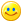(Strife would probably write llVecDist(lastpos, lastpos=llGetPos()); which probably works now, but in my mind qualifies as teasing the foulup fairy)